## What is the value of delta G at 292 for a reaction in which deltaH = 92 kj/mol and delta S= 2.38 kj/mol K

Question

What is the value of delta G at 292 for a reaction in which deltaH = 92 kj/mol and delta S= 2.38 kj/mol K

in progress 0
6 months 2021-08-10T05:45:02+00:00 1 Answers 3 views 0

## Answers ( )

1. Answer: The value of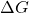is -602.96 kJ/mol at 292 k for a reaction in which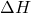= 92 kj/mol and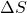= 2.38 kj/mol K.

Explanation:

Given: Temperature = 292 K= 92 kJ/mol= 2.38 kJ/mol

Formula used to calculate the value ofis as follows.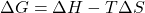Substitute the values into above formula as follows.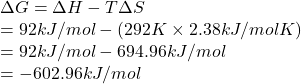Thus, we can conclude that the value ofis -602.96 kJ/mol at 292 k for a reaction in which= 92 kj/mol and= 2.38 kj/mol K.